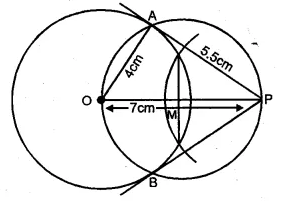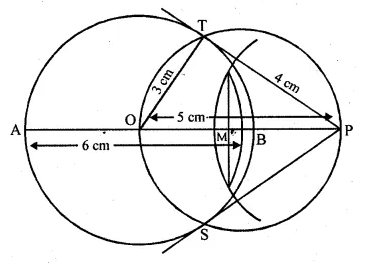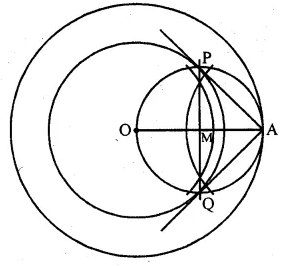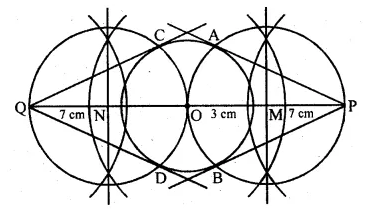## ML Aggarwal Class 10 Solutions for ICSE Maths Chapter 16 Constructions Ex 16.1

These Solutions are part of ML Aggarwal Class 10 Solutions for ICSE Maths. Here we have given ML Aggarwal Class 10 Solutions for ICSE Maths Chapter 16 Constructions Ex 16.1 More Exercises

Question 1. Use a ruler and compass only in this question. (i) Draw a circle, centre O and radius 4 cm. (ii) Mark a point P such that OP = 7 cm. Construct the two tangents to the circle from P. Measure and record the length of one of the tangents. Solution: Steps of Construction:

1. Draw a circle with centre O and radius 4 cm.
2. Take a point P such that OP = 7 cm.
3. Bisect OB at M.
4. With centre M and diameter OP, draw another circle intersecting the given circle at A and B.
5. Join PA and PB. PA and PB is a pair of tangents to the circle.
6. On measuring PA, it is equal to 5.5 cm.Question 2. Draw a line AB = 6 cm. Construct a circle with AB as diameter. Mark a point P at a distance of 5 cm from the mid-point of AB. Construct two tangents from P to the circle with AB as diameter. Measure the length of each tangent Solution: Steps of Construction:

1. Take a line segment AB = 6 cm.
2. Draw its perpendicular bisector bisecting it at O.
3. With centre O and radius OB, draw a circle.
4. Produce AB to P such that OP = 5 cm.
5. Draw its perpendicular bisector intersecting it at M.
6. With centre M and radius OM, draw a circle which intersects the given circle at T and S.
7. Join OT, OS, TP and SP. PT and PS are the required tangents to the given circle.
8. On measuring, each tangent is 4 cm long i.e. PT = PS = 4 cm.Question 3. Construct a tangent to a circle of radius 4cm from a point on the concentric circle of radius 6 cm and measure its length. Also, verify the measurement by actual calculation. Solution: Steps of construction:

1. Take a point O.
2. With centre O and radii 4 cm and 6 cm, draw two concentric circles.
3. Join OA and take its mid-point M.
4. With centre M and radius MA, draw another circle which intersects the first circle at P and Q.
5. Join AP and AQ. AP and AQ are the required tangents to the first circle from point A.Question 4. Draw a circle of radius 3 cm. Take two points P and Q on one of its extended diameter each at a distance of 7 cm from its centre. Draw tangents to the circle from these two points P and Q. Solution: Steps of construction:

1. Take a point O and with centre O, and radius 3 cm, draw a circle.
2. Produce its diameter both sides and cut off OP = OQ = 7 cm.
3. Take mid-points of OP and OQ as M and N respectively.
4. With centres M and N and OP and OQ as diameters, draw circles which intersect the given circle at A, B and C and D respectively.
5. Join PA, PB, QC and QD. PA, PB and QC and QD are the required tangents.Question 5. Draw a line segment AB of length 8 cm. Taking A as centre, draw a circle of radius 4 cm and taking B as centre, draw another circle of radius 3 cm. Construct tangents to each circle from the centre of the other circle. Solution: Steps of construction:

1. Draw a line segment AB = 8 cm.
2. With centre A and radius 4 cm and with centre B and radius 3 cm, draw circles.
3. Draw a third circle AB as diameter which intersects the given two circles at C and D and P and Q respectively.
4. Join AC and AD, BP and BQ.
5. AC and AD, BP and BQ are the required tangents.Hope given ML Aggarwal Class 10 Solutions for ICSE Maths Chapter 16 Constructions Ex 16.1 are helpful to complete your math homework. If you have any doubts, please comment below. Learn Insta try to provide online math tutoring for you.